# Control of highly anisotropic electrical conductance of tellurene by strain-engineering†

Huanhuan Ma, Wei Hu* and Jinlong Yang*
Hefei National Laboratory for Physical Sciences at Microscale, Department of Chemical Physics, and Synergetic Innovation Center of Quantum Information and Quantum Physics, University of Science and Technology of China, Hefei, Anhui 230026, China. E-mail: whuustc@ustc.edu.cn; jlyang@ustc.edu.cn

Received 4th July 2019 , Accepted 11th October 2019

First published on 14th October 2019

Tailoring the electronic anisotropy of two-dimensional (2D) semiconductors with strain-engineering is critical in nanoelectronics. Recently, 2D tellurene has been predicted theoretically and fabricated experimentally. It has potential applications in nanoelectronics, in particular, β-phase tellurene (β-Te) shows a desirable direct band gap (1.47 eV), high carrier mobility (2.58 × 103 cm2 V−1 s−1) and high stability under ambient conditions. In this work, we demonstrated, with first-principles density functional theory calculations, that the highly anisotropic electron mobility and electrical conductance of β-Te can be controlled by strain-engineering. The direction of electrical conductance of β-Te can be changed from the armchair to the zigzag direction at the strain between −1% and 0%. Meanwhile, we found that the bandgap of β-Te under strain experiences an indirect-direct transition with a conduction band minimum (CBM) shift from the X to Γ point. The significant dispersion of the bottom of the conduction bands along the ΓY direction switches to the XΓ direction under uniaxial or biaxial strain which makes the rotation of the effective masses tensor. The qualitative rotation of the spatial anisotropic electron effective masses tensor by 90° also rotates the direction of the electrical conduction as the carrier mobility is inversely dependent on the effective masses. On the another hand, we also found that the deformation potential constant also plays an important role in the rotation of electrical conductance anisotropy. While anisotropic conductance of hole is impregnable under strain. In order to verify that β-Te can sustain large strain, we studied its stability and mechanical properties and found that β-Te shows superior mechanical flexibility with a small Young's modulus (27.46 GPa (armchair)–61.99 GPa (zigzag)) and large anisotropic strain-stress (12.89 N m−1 at the strain of 38% along armchair direction and 25.72 N m−1 at the strain of 26% along zigzag direction). The high anisotropic carrier mobility and superior mechanical flexibility of β-Te make it a promising candidate for flexible nanoelectronics.

## 1. Introduction

Since graphene was fabricated by Novoselov and Geim,1 many two dimensional (2D) monolayers have been synthesized over the past decades, including phosphorene,2,3 transition metal dichalcogenides (TMDs),4–6 hexagonal boron nitride (h-BN),7–9 graphitic carbon nitride (g-C3N4)10,11 and borophene.12 2D materials have attracted great attention because of their many fascinating electronic, optical, thermal and mechanical properties.13,14 In particular, tailoring the optical and electronic anisotropy of 2D semiconductors with external experimental techniques, such as electric fields15,16 and strain-engineering,17,18 is critical for their application in photocatalysis19–21 and nanoelectronics.15,18,22,23 However, the anisotropic properties of most 2D semiconductors can only be quantitatively modulated by external experimental techniques, such as electric fields and strain engineering. In particular, the anisotropic electrical conductance of 2D semiconductors can only be quantitatively enhanced or weakened under strain,18,24 that is only the values of the carrier mobility can be changed, but the direction of conductance can rarely be qualitatively modulated.22,25

On the other hand, the electronic properties of most traditional semiconductors are poor under large strain, which makes it difficult to apply them directly in flexible electronics.26 Therefore, the search is urgent for promising semiconductors with desirable electronic and mechanical properties when they are under strain. Flexibility is a physical quantity which describes the ability of materials to resist deformation in response to an applied force, and is an important factor in flexible electronics. Compared with bulk materials, the counterparts of nanostructures can withstand larger strain and bending,27–29and have potential applications in nanoelectronics. Thus, we search for promising 2D semiconductors for use in flexible nanoelectronics. To develop potential flexible nanoelectronics, 2D semiconductors must have some essential properties. Firstly, 2D materials must have a suitable bandgap and a high carrier mobility. Secondly, semiconductors can withstand room temperature and ambient conditions. However, for flexible electronic devices, 2D materials must sustain large strain. Unfortunately, despite the fact that a large number of 2D materials have been synthesized, there is a scarcity of materials which meet all of these properties. For example, graphene is stable under ambient conditions and has a high carrier mobility,30 but doesn't have a bandgap.14 MoS2 is stable under ambient conditions31 and has a suitable bandgap,32 but the Young's modulus is medium33 and the carrier mobility is too low.34 Black phosphorene has a small Young's modulus,35 a suitable bandgap, highly anisotropic carrier mobility and strain-engineered anisotropic electrical conductance,3,22 but is unstable under ambient conditions.36

2D tellurene has recently been predicted theoretically and fabricated experimentally.37,38 Tellurene has three different structures with different electronic properties. α-phase tellurene (α-Te) and β-phase tellurene (β-Te) are both semiconductors with an indirect bandgap of 0.75 eV and a direct bandgap of 1.47 eV, respectively; while γ-phase tellurene (γ-Te) is a metal.37 The carrier mobilities and the on/off ratio of field-effect transistors made from a few layers 2D tellurene were found to be 700 cm2 V−1 s−1 and in the order of 106 respectively, in recent experiment.38 In addition, air-stable quasi-2D tellurium nanoflakes were synthesized and used as short-wave infrared photodetectors in a previous experiment.39 Tellurene has also been found to show low lattice thermal conductivity,40 excellent thermoelectric performance41–43 and high thermal and chemical stability under ambient conditions.38,44 These results may make tellurene a promising candidate material for electronic, optoelectronic and thermoelectric devices. Therefore, a further study of the strain effect on electronic and mechanical properties of tellurene should be helpful to exploit its potential application in flexible electronics.

In this work, we examined the electronic and mechanical anisotropy of tellurene by using first-principles density functional theory calculations. We found that strain-engineering can be used to control the anisotropic electrical conductance of β-Te. Moreover, we demonstrated the strain effect on the carrier mobilities of β-Te by using the acoustic phonon limited method. The mobilities of holes and electrons have different responses to strain, which can control the direction of electrical conductance. We also explored the intrinsic mechanism of the anisotropic electron mobility of β-Te under strain. We showed the strain effect on the effective masses tensor and band structure of β-Te. The effective mass of the electrons of intrinsic β-Te in the zigzag direction is an order of magnitude smaller than that in the armchair direction, indicating that the zigzag direction is favored for electron transport. Furthermore, strain can rotate the spatial anisotropic effective masses tensor, which can be used to control the electrical conductance as the carrier mobility is inversely dependent on the effective mass. Finally, in order to verify that β-Te can sustain large strain, we studied the stability and mechanical properties of β-Te. β-Te has the smallest Young's modulus (27.46 GPa) and the largest elastic strain limit (38%) than most common 2D semiconductors and shows potential application in flexible devices.

## 2. Methodology

First-principles density functional theory (DFT)45 calculations were performed in the Vienna ab initio simulation package (VASP).46 Structure relaxations and self-consistent total energy calculations were simulated by using the generalized gradient approximation of Perdew, Burke, and Ernzerhof (GGA-PBE)47 exchange–correlation functional along with the projector-augmented wave (PAW) potentials.48 For all structural relaxations, the convergence criterion for the energy in electronic self consistent field (SCF) iterations and the Hellmann–Feynman force in ionic step iterations were set to be 10−6 eV and 10−3 eV Å−1, respectively. The reciprocal space was meshed using the 10 × 12 × 1 Monkhorst–Pack method for β-Te. In order to reduce the interaction between neighboring layers, a large vacuum space of at least 15 Å was introduced along the z-axis. The van der Waals (vdW) correction proposed by Grimme (DFT-D2)49 was used in our calculations. We also used Phonopy50,51 to calculate the phonon spectrum.

By using the acoustic phonon limited method,52,53 we calculated the mobilities of α-Te and β-Te under strain,

 (1)
where e and ħ are the electron charge and reduced Planck constant, respectively. The temperature T was chosen to be 300 K, kB is the Boltzmann constant, m* and md are the effective mass and the average effective mass, respectively.

We used the finite distortion method to calculate the mechanical properties of 2D materials, such as elastic constants, Young's modulus and Poisson's ratio. For 2D materials, the elastic constants and modulus were obtained from the Hooke's law under plane-stress conditions54

 (2)
where C11, C12, C22, and C66 are the elastic stiffness constants. Then, the orientation-dependent Young's modulus E(θ) and Poisson's ratio υ(θ) were calculated as follows:55
 (3)
where d1, d2, d3, Yzz and υzz are all elastic constants (see ESI).

For 2D materials, the stress was calculated using the Hellmann–Feynman theorem, modified to be the force per unit length.56 Tensile strain was applied to the chosen crystalline direction, and the corresponding stress was calculated. For 2D materials, the tensile stress σ is given by

 (4)
where Etotal is the strain energy, and S(εij) is the area under the given tensile strain. The εij denotes the tensile strain of the simulation cell, which is defined as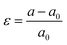, where a and a0 are lattice constants of the strained and relaxed structure, respectively.

## 3. Results and discussion

### 3.1. Geometrical and electronic properties of β-Te

We first checked the geometrical and electronic properties of β-Te. We found that the symmetry of β-Te is P2/m, as shown in Fig. 1(a), and the lattice parameters are a = 5.49 Å and b = 4.17 Å, respectively, which are consistent with previous theoretical works.37 Two-layer β-Te has a sequence of AB stacking as bulk tellurium. The three- and four-layer β-Te structure has a stacking sequence of ABA and ABAB, respectively and the mean interlayer distance of β-Te is 1.80 Å (see ESI). We also checked the electronic properties of tellurene. The PBE + SOC band structure of β-Te is presented in Fig. 1(b). β-Te is a semiconductor with a direct bandgap of 1.03 eV within the PBE + SOC scheme (1.47 eV with the HSE + SOC scheme, see ESI), meanwhile the bandgap as a function of the number of layers for tellurene was also calculated (see ESI). Using the acoustic phonon limited method52,53 we calculated the smallest effective mass and the highest carrier mobility of β-Te (see ESI), the values were found to be 0.11me and 2.58 × 103 cm2 V−1 s−1, respectively, and are consistent with previous theoretical work.37,57 It should be noted that the relatively small effective mass and high carrier mobility of tellurene mean that it is of potential use in nanoelectronic devices.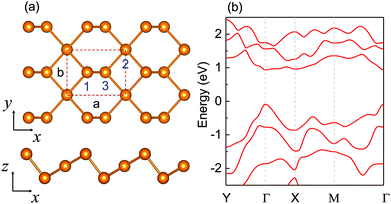Fig. 1 (a) Top and side views of β-Te, and (b) band structure of β-Te within the PBE + SOC scheme.

### 3.2. Carrier mobilities of β-Te under strain

We then calculated the electron and hole mobilities of β-Te at room temperature (T = 300 K) by using eqn (2) according to biaxial strain, armchair uniaxial strain and zigzag uniaxial strain as shown in Fig. 2. We found that electron mobility along the armchair direction is significantly improved when the biaxial, armchair and zigzag compressed strain is applied, but electron mobility along th zigzag direction increased gradually under compressed uniaxial or biaxial strain(expect for −6% strain along zigzag direction), as shown in Fig. 2(a), (b) and (c). On the other hand, there was a slight change in the electron mobility when tensile strain was applied. Meanwhile, we observed that the anisotropic electron mobilities have a critical transition at the strain between −1% and 0%, which can be well confirmed in experiments. When applying the 6% compressed biaxial, uniaxial strain along the armchair and zigzag direction, on β-Te, the mobility of electrons along the armchair direction was around 19, 7.6 and 8 times of magnitude larger than that along the zigzag direction, respectively. But, when applying the +5% tensile biaxial, uniaxial strain along the armchair and zigzag direction, the mobility of electrons along the zigzag direction was around 3.6, 5 and 9 times of magnitude larger than that along the armchair direction, respectively. Therefore, strain-engineering in β-Te can switch the direction of anisotropic electrical conductance, as in the case of black phosphorene (α-P)22,25 and other phosphorene allotropes (γ-P).25 This is a common phenomenon in some phosphorene allotropes and seems to be common in other group-V allotropes.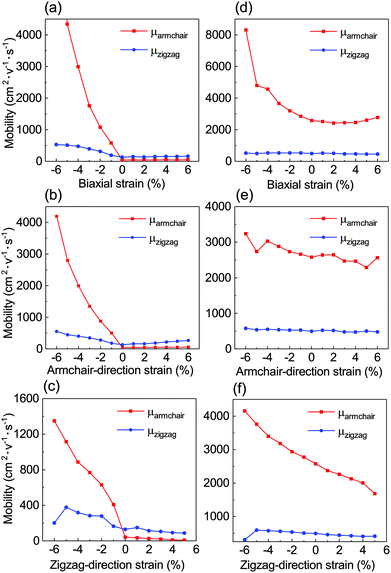Fig. 2 Electron mobilities of β-Te along armchair (μarmchair) and zigzag (μzigzag) directions for (a) biaxial strain, (b) uniaxial armchair-direction (x) strain and (c) uniaxial zigzag-direction (y) strain at room temperature (T = 300 K), respectively. Hole mobilities of β-Te along armchair (μarmchair) and zigzag (μzigzag) directions for (d) biaxial strain, (e) uniaxial armchair-direction (x) strain and (f) uniaxial zigzag-direction (y) strain at room temperature (T = 300 K), respectively.

On the other hand, the hole mobility along the armchair direction was significantly improved when biaxial and zigzag direction compressed strain were applied as shown in Fig. 2(d) and (e), but was slightly changed under armchair direction strain from Fig. 2(f). At the same time, hole mobility along the zigzag direction was almost constant under uniaxial or biaxial strain (expect for the −6% strain along zigzag direction). So, the strain effect can just enhance or weaken the conductance of the hole, but cannot change the direction.

### 3.3. Intrinsic mechanism of anisotropic electrical conductance of β-Te under strain

In order to explore the rotation of anisotropic conductance of β-Te, the electron effective masses of β-Te were calculated under biaxial strain as shown in Fig. 3. The intrinsic electron effective mass of β-Te was found to be anisotropic and the values differed by an order of magnitude as illustrated in the real space in Fig. 3(a). The effective mass was 1.1me along the armchair direction and 0.2me along the zigzag direction. Compared with Fig. 3(a), the anisotropic effective mass of electrons was rotated by exactly 90° in Fig. 3(b) for the 6% biaxially compressed β-Te. The electrons have a small effective mass along the zigzag direction and a large effective mass along the armchair direction when β-Te is under biaxially tensile strain (see ESI). The rotation occurs when β-Te is under the biaxially compressed strain resulting in rotation of electron mobility as the carrier mobility is inversely dependent on the effective masses. In addition to the biaxial strain, a similar rotation of the electron effective mass tensor was observed under uniaxial strain along the zigzag and armchair direction of β-Te.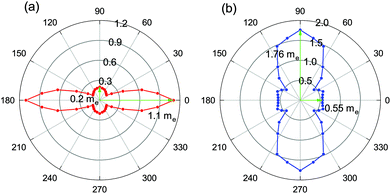Fig. 3 Electron effective masses tensor according to spatial direction of (a) intrinsic β-Te and (b) 6% biaxially compressed β-Te. The length of blue arrow represents absolute values of effective mass.

As discussed in section 2, in addition to the influence of effective mass on the carrier mobility, two other parameters (elastic modulus (C2D) and deformation potential constant (E1i)) can also influence the mobility of electrons. As we see from Fig. S14(a), the ratio of elastic modulus in the zigzag and armchair direction is changed slightly, so elastic modulus can slightly influence electronic mobility. On the other hand, we found that the ratio of the deformation potential constant of electrons along the zigzag and armchair directions was similar to that presented in Fig. S24(b), so the deformation potential constant also plays an important role in the rotation of anisotropy.

It should be noted that the electrical transport behaviour in semiconductors is mainly dependent on the band edges of valence and conduction states, including the valence band maximum (VBM) and the conduction band minimum (CBM). The VBM and CBM of intrinsic β-Te respectively are determined by the py and px orbitals of Te at the Γ point, which can be found from the projected band structure (pband) of intrinsic β-Te and can also be seen from the projected density of states, pDOS (see ESI). When a −6% or +6% biaxial strain was applied to β-Te, there was almost no change in the VBM and it was still mainly determined by the py orbitals of Te at the Γ point as shown in Fig. 4(a) and (b). In particular, when the −6% biaxial strain was applied in β-Te, the CBM shifted from Γ to X (0.5, 0, 0) and β-Te became an indirect semiconductor as shown in Fig. 4(a), which was validated by using the HSE06 + SOC (see ESI). In this case, the CBM is mainly contributed by the pz orbitals of Te at X point because two Te atoms become close to each other, resulting in pz orbital overlap between two Te atoms, and pz orbit is contributed from the two outer-layer Te atoms (1 and 3 in Fig. 1(a), see ESI). While the +6% biaxial strain is applied to β-Te, the CBM almost has no change and is still mainly contributed by the px orbitals of Te at Γ point as shown in Fig. 4(b). The contribution of the pz orbital of the outer-later Te atom will increase when the compressed strain was applied to β-Te, but the contribution decreased when tensile strain was applied (see ESI). This is the main intrinsic mechanism of the anisotropic electron mobilities of β-Te.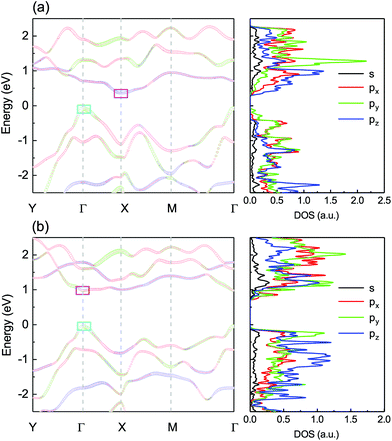Fig. 4 Projected band structure (pband) and density of states (pDOS) of β-Te under (a) −6% and (b) +6% biaxial strain. Fermi levels are set to zero. The box of cyan and magenta represent VBM and CBM, respectively.

In addition to the shift of CBM, another important feature of +6% biaxial strained β-Te is its highly anisotropic band dispersion around the CBM. The bottom of the conduction bands have much more significant dispersions along the ΓY direction, which is the zigzag direction in real space; however, along the ΓX direction it is nearly flat, which is the armchair direction in real space. Such similar phenomenon can also be found in intrinsic β-Te. But when −6% strain is applied to β-Te rotation occurs, therefore, the conduction bands along the XM direction are relatively flat compared to those along the XΓ direction. This can also be confirmed by the effective mass of biaxially strained β-Te.

### 3.4. Stability and mechanical properties of β-Te

In order to verify that β-Te can sustain large strain, we also studied its stability and mechanical properties. Elastic properties were used to evaluate the interatomic bonding strength and lattice vibration anharmonicity. We evaluated the elastic properties from the interatomic bonding strength and lattice vibration anharmonicity in turn. As is known, the lattice vibration anharmonicity is smaller than harmonicity in most covalent materials, so the elastic properties can be evaluated from the phonon spectrum. We first calculated the phonon spectrum of β-Te, which is shown in Fig. 5(a). The phonon spectrum can not only be used to evaluate the mechanical properties by the longitudinal (LA) mode and transverse (TA) mode of acoustic phonon dispersions around the Γ point,58 but can also be used to show the stability of 2D materials. For comparison, we also calculated the phonon spectra of graphene, MoS2 and phosphorene (see ESI). The heights of the acoustic phonon dispersions were 1326.2 cm−1, 231.0 cm−1 and 193.1 cm−1 for graphene, MoS2 and phosphorene, respectively, which are consistent with previous theoretical works.59–61 It should be noted that the Young's modulus of graphene is the largest and that of phosphorene is the lowest of the materials studied.33,35,62 While we found that the height of acoustic phonon dispersions was 69.9 cm−1 for β-Te. As expected, the Young's modulus of α-Te and β-Te were small.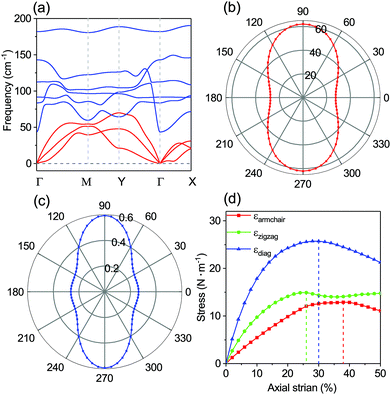Fig. 5 The orientation-dependent (a) phonon band structure of β-Te, where red represents acoustic branch and blue represents optical branch of phonon, respectively. (b) Young's modulus E(θ) in GPa, (c) Poisson's ratio silon(θ) of β-Te, respectively. (d) The stress as a function of tensile load for β-Te.

Using the orientation-dependent relation, the polar diagrams of Young's modulus E(θ) and Poisson's ratio υ(θ) for β-Te computed are plotted in Fig. 5(b) and (c). The Young's modulus represents the fully reversible stiffness response as in a linear elastic Hookean spring.65 As expected, the Young's modulus and Poisson's ratio of β-Te are highly anisotropic in Fig. 5(b) and (c). The maximal Young's modulus of 61.99 GPa is along the zigzag direction and the minimal value of 27.46 GPa is along the armchair direction of β-Te, which are consistent with previous theoretical work.66 But for multilayer β-Te, the maximal Young's modulus is along the armchair direction and the minimum is along the zigzag direction, because the structures of multilayer β-Te are similar to the bulk (see ESI). We found that β-Te has the lowest Young's modulus compared to that of graphene,63,67 MoS264 and phosphroene,35 as presented in Table 1.

Table 1 Bandgap Eg (eV), carrier mobility μ (103 cm2 V−1 s−1), Young's modulus E (GPa), elastic strain limit ε and ambient stability of graphene, phosphorene, MoS2, α-Te and β-Te
2D materials Eg μ E ε Stability
Graphene30,62,63 0 100 1000 30% High
MoS234,64 1.64 0.34 330 26% High
phosphorene17,22,35,36 1.61 2.2 44 30% Low
α-Te 0.75 4.06 57.96 20% High
β-Te 1.47 2.58 27.46 38% High

To further explore the mechanical properties of tellurene, the ideal tensile strength (the highest achievable stress of a perfect crystal at 0 K) and the critical strain (the strain at which ideal strength reaches)68 of β-Te were also studied. An in-plane tensile force was applied along the armchair, zigzag or diagonal direction to research the strain-stress relation. Using eqn (4), our calculated stress–strain relation is presented in Fig. 5(d) for β-Te. The applied strain ranges from 0 to 50% for β-Te. It shows that the ideal strengths of β-Te are up to 12.89 N m−1, 14.92 N m−1 and 25.72 N m−1 in the armchair, zigzag and diagonal direction, respectively. The corresponding tensile strain limits are 38%, 26% and 30% along the armchair, zigzag, and diagonal directions, respectively, that's because β-Te has a puckered structure which can be seen from Fig. 1(a). This predicted elastic strain limit is larger than that of other 2D materials such as graphene,62,69 MoS270 and phosphorene,17 suggesting that tellurene is highly flexible and may have potential applications in flexible devices.

## 4. Conclusions

In summary, we demonstrated by first-principles density functional theory calculations that the anisoitropic electrical conductance of β-Te can be controlled by external strain. Using the acoustic phonon limited method, β-Te was determined to have high carrier mobilities of up to 2.58 × 103 cm2 V−1 s−1. The mobility of electrons along thearmchair direction was almost an order of magnitude larger than that along the zigzag direction, when the 6% compressed zigzag strain was applied. But the mobility of electrons along the zigzag direction was almost an order of magnitude larger than that along the armchair direction, when the 5% tensile zigzag strain was applied. Meanwhile, the mobility of the hole can only be enhanced or weakened under strain. We found that the anisotropic electron effective masses tensor and corresponding mobility direction of β-Te can be rotated by 90° by applying uniaxial or biaxial strain, while its anisotropic holes were impregnable. The rotation was confirmed by the band structure of β-Te under strain. Finally, in order to verify that β-Te can sustain large strain, we studied its stability and mechanical properties. This work reveals that β-Te has high anisotropic carrier mobility, strain-engineered anisotropic conductance and superior mechanical flexibility, which means that it will be useful in the design of stretchable electrical devices, mechanically controlled logical devices, and nanosized mechanical sensors.71

## Conflicts of interest

There are no conflicts of interest to declare.

## Acknowledgements

This work is supported by National Natural Science Foundation of China (21503204, 21688102 and 21803066), by National Key Research and Development Program of China (2016YFA0200604), Anhui Initiative in Quantum Information Technologies (AHY090400), Strategic Priority Research Program of Chinese Academy of Sciences (XDC01040100), Research Start-Up Grants (KY2340000094) and Academic Leading Talents Training Program (KY2340000103) from University of Science and Technology of China, and Chinese Academy of Sciences Pioneer Hundred Talents Program. The authors thank USTC, CAS, Tianjin, and Shanghai Supercomputer Centers for the computational resources.

## References

1. K. S. Novoselov, A. K. Geim, S. V. Morozov, D. Jiang, Y. Zhang, S. V. Dubonos, I. V. Grigorieva and A. A. Firsov, Science, 2004, 306, 666–669 CrossRef CAS PubMed.
2. L. Li, Y. Yu, G. J. Ye, Q. Ge, X. Ou, H. Wu, D. Feng, X. H. Chen and Y. Zhang, Nat. Nanotechnol., 2014, 9, 372 CrossRef CAS PubMed.
3. H. Liu, A. T. Neal, Z. Zhu, Z. Luo, X. Xu, D. Tománek and P. D. Ye, ACS Nano, 2014, 8, 4033–4041 CrossRef CAS PubMed.
4. A. Splendiani, L. Sun, Y. Zhang, T. Li, J. Kim, C.-Y. Chim, G. Galli and F. Wang, Nano Lett., 2010, 10, 1271–1275 CrossRef CAS PubMed.
5. G. Eda, H. Yamaguchi, D. Voiry, T. Fujita, M. Chen and M. Chhowalla, Nano Lett., 2011, 11, 5111–5116 CrossRef CAS PubMed.
6. H. Ramakrishna Matte, A. Gomathi, A. K. Manna, D. J. Late, R. Datta, S. K. Pati and C. Rao, Angew. Chem., Int. Ed., 2010, 49, 4059–4062 CrossRef PubMed.
7. K. Watanabe, T. Taniguchi and H. Kanda, Nat. Mater., 2004, 3, 404 CrossRef CAS PubMed.
8. L. Song, L. Ci, H. Lu, P. B. Sorokin, C. Jin, J. Ni, A. G. Kvashnin, D. G. Kvashnin, J. Lou and B. I. Yakobson, et al., Nano Lett., 2010, 10, 3209–3215 CrossRef CAS PubMed.
9. K. K. Kim, A. Hsu, X. Jia, S. M. Kim, Y. Shi, M. Hofmann, D. Nezich, J. F. Rodriguez-Nieva, M. Dresselhaus and T. Palacios, et al., Nano Lett., 2011, 12, 161–166 CrossRef PubMed.
10. X. Wang, K. Maeda, X. Chen, K. Takanabe, K. Domen, Y. Hou, X. Fu and M. Antonietti, J. Am. Chem. Soc., 2009, 131, 1680–1681 CrossRef CAS PubMed.
11. A. Thomas, A. Fischer, F. Goettmann, M. Antonietti, J.-O. Müller, R. Schlögl and J. M. Carlsson, J. Mater. Chem., 2008, 18, 4893–4908 RSC.
12. A. J. Mannix, X.-F. Zhou, B. Kiraly, J. D. Wood, D. Alducin, B. D. Myers, X. Liu, B. L. Fisher, U. Santiago and J. R. Guest, et al., Science, 2015, 350, 1513–1516 CrossRef CAS PubMed.
13. C. Tan, X. Cao, X.-J. Wu, Q. He, J. Yang, X. Zhang, J. Chen, W. Zhao, S. Han and G.-H. Nam, et al., Chem. Rev., 2017, 117, 6225–6331 CrossRef CAS PubMed.
14. S. D. Sarma, S. Adam, E. Hwang and E. Rossi, Rev. Mod. Phys., 2011, 83, 407 CrossRef.
15. Q. Liu, X. Zhang, L. Abdalla, A. Fazzio and A. Zunger, Nano Lett., 2015, 15, 1222–1228 CrossRef CAS PubMed.
16. J. Kim, S. S. Baik, S. H. Ryu, Y. Sohn, S. Park, B.-G. Park, J. Denlinger, Y. Yi, H. J. Choi and K. S. Kim, Science, 2015, 349, 723–726 CrossRef CAS PubMed.
17. X. Peng, Q. Wei and A. Copple, Phys. Rev. B: Condens. Matter Mater. Phys., 2014, 90, 085402 CrossRef.
18. M. Zhou, X. Chen, M. Li and A. Du, J. Mater. Chem. C, 2017, 5, 1247–1254 RSC.
19. B. Sa, Y.-L. Li, J. Qi, R. Ahuja and Z. Sun, J. Phys. Chem. C, 2014, 118, 26560–26568 CrossRef CAS.
20. X. Li, Z. Li and J. Yang, Phys. Rev. Lett., 2014, 112, 018301 CrossRef PubMed.
21. C.-F. Fu, J. Sun, Q. Luo, X. Li, W. Hu and J. Yang, Nano Lett., 2018, 18, 6312–6317 CrossRef CAS PubMed.
22. R. Fei and L. Yang, Nano Lett., 2014, 14, 2884–2889 CrossRef CAS PubMed.
23. Y.-C. Lin, H.-P. Komsa, C.-H. Yeh, T. Bjorkman, Z.-Y. Liang, C.-H. Ho, Y.-S. Huang, P.-W. Chiu, A. V. Krasheninnikov and K. Suenaga, ACS Nano, 2015, 9, 11249–11257 CrossRef CAS PubMed.
24. W. Zhang, J. Yin, Y. Ding, Y. Jiang and P. Zhang, Nanoscale, 2018, 10, 16750–16758 RSC.
25. A. Priydarshi, Y. S. Chauhan, S. Bhowmick and A. Agarwal, Phys. Rev. B, 2018, 97, 115434 CrossRef CAS.
26. Y. Liu, K. He, G. Chen, W. R. Leow and X. Chen, Chem. Rev., 2017, 117, 12893–12941 CrossRef CAS PubMed.
27. X. Peng and P. Logan, Appl. Phys. Lett., 2010, 96, 143119 CrossRef.
28. A. I. Hochbaum and P. Yang, Chem. Rev., 2009, 110, 527–546 CrossRef PubMed.
29. G. Van Lier, C. Van Alsenoy, V. Van Doren and P. Geerlings, Chem. Phys. Lett., 2000, 326, 181–185 CrossRef CAS.
30. E. Hwang and S. D. Sarma, Phys. Rev. B: Condens. Matter Mater. Phys., 2008, 77, 115449 CrossRef.
31. D. J. Late, B. Liu, H. R. Matte, V. P. Dravid and C. Rao, ACS Nano, 2012, 6, 5635–5641 CrossRef CAS PubMed.
32. T. Cheiwchanchamnangij and W. R. Lambrecht, Phys. Rev. B: Condens. Matter Mater. Phys., 2012, 85, 205302 CrossRef.
33. S. Bertolazzi, J. Brivio and A. Kis, ACS Nano, 2011, 5, 9703–9709 CrossRef CAS PubMed.
34. Y. Cai, G. Zhang and Y.-W. Zhang, J. Am. Chem. Soc., 2014, 136, 6269–6275 CrossRef CAS PubMed.
35. Q. Wei and X. Peng, Appl. Phys. Lett., 2014, 104, 251915 CrossRef.
36. A. Favron, E. Gaufrès, F. Fossard, A.-L. Phaneuf-LHeureux, N. Y. Tang, P. L. Lévesque, A. Loiseau, R. Leonelli, S. Francoeur and R. Martel, Nat. Mater., 2015, 14, 826 CrossRef CAS PubMed.
37. Z. Zhu, X. Cai, S. Yi, J. Chen, Y. Dai, C. Niu, Z. Guo, M. Xie, F. Liu and J.-H. Cho, et al., Phys. Rev. Lett., 2017, 119, 106101 CrossRef PubMed.
38. Y. Wang, G. Qiu, R. Wang, S. Huang, Q. Wang, Y. Liu, Y. Du, W. A. Goddard, M. J. Kim and X. Xu, et al., Nat. Electron., 2018, 1, 228 CrossRef.
39. M. Amani, C. Tan, G. Zhang, C. Zhao, J. Bullock, X. Song, H. Kim, V. R. Shrestha, Y. Gao and K. B. Crozier, et al., ACS Nano, 2018, 12, 7253–7263 CrossRef CAS PubMed.
40. Z. Gao, F. Tao and J. Ren, Nanoscale, 2018, 10, 12997–13003 RSC.
41. S. Sharma, N. Singh and U. Schwingenschlogl, ACS Appl. Energy Mater., 2018, 1, 1950–1954 CrossRef CAS.
42. C. Lin, W. Cheng, G. Chai and H. Zhang, Phys. Chem. Chem. Phys., 2018, 20, 24250–24256 RSC.
43. Z. Gao, G. Liu and J. Ren, ACS Appl. Mater. Interfaces, 2018, 10, 40702–40709 CrossRef CAS PubMed.
44. X. H. Wang, D. W. Wang, A. J. Yang, N. Koratkar, J. F. Chu, P. L. Lv and M. Z. Rong, Phys. Chem. Chem. Phys., 2018, 20, 4058–4066 RSC.
45. W. Kohn and L. J. Sham, Phys. Rev., 1965, 140, A1133 CrossRef.
46. G. Kresse and J. Furthmüller, Phys. Rev. B: Condens. Matter Mater. Phys., 1996, 54, 11169 CrossRef CAS PubMed.
47. J. P. Perdew, K. Burke and M. Ernzerhof, Phys. Rev. Lett., 1996, 77, 3865 CrossRef CAS PubMed.
48. P. E. Blöchl, Phys. Rev. B: Condens. Matter Mater. Phys., 1994, 50, 17953 CrossRef PubMed.
49. S. Grimme, J. Comput. Chem., 2006, 27, 1787–1799 CrossRef CAS PubMed.
50. A. Togo, F. Oba and I. Tanaka, Phys. Rev. B: Condens. Matter Mater. Phys., 2008, 78, 134106 CrossRef.
51. A. Togo and I. Tanaka, Scr. Mater., 2015, 108, 1–5 CrossRef CAS.
52. J. Bardeen and W. Shockley, Phys. Rev., 1950, 80, 72 CrossRef CAS.
53. S. Bruzzone and G. Fiori, Appl. Phys. Lett., 2011, 99, 222108 CrossRef.
54. J. Zhou and R. Huang, J. Mech. Phys. Solids, 2008, 56, 1609–1623 CrossRef CAS.
55. L. Wang, A. Kutana, X. Zou and B. I. Yakobson, Nanoscale, 2015, 7, 9746–9751 RSC.
56. J. Hudson, Surface science: an introduction, Elsevier, 2013 Search PubMed.
57. K. Wu, H. Ma, Y. Gao, W. Hu and J. Yang, J. Mater. Chem. A, 2019, 7, 7430–7436 RSC.
58. Y. Xiao, C. Chang, Y. Pei, D. Wu, K. Peng, X. Zhou, S. Gong, J. He, Y. Zhang and Z. Zeng, et al., Phys. Rev. B, 2016, 94, 125203 CrossRef.
59. L. Lindsay and D. Broido, Phys. Rev. B: Condens. Matter Mater. Phys., 2010, 81, 205441 CrossRef.
60. Y. Cai, J. Lan, G. Zhang and Y.-W. Zhang, Phys. Rev. B: Condens. Matter Mater. Phys., 2014, 89, 035438 CrossRef.
61. A. Jain and A. J. McGaughey, Sci. Rep., 2015, 5, 8501 CrossRef CAS PubMed.
62. C. Lee, X. Wei, J. W. Kysar and J. Hone, Science, 2008, 321, 385–388 CrossRef CAS.
63. B. Hajgato, S. Guryel, Y. Dauphin, J.-M. Blairon, H. E. Miltner, G. Van Lier, F. De Proft and P. Geerlings, J. Phys. Chem. C, 2012, 116, 22608–22618 CrossRef CAS.
64. A. Castellanos-Gomez, M. Poot, G. A. Steele, H. S. van der Zant, N. Agraït and G. Rubio-Bollinger, Adv. Mater., 2012, 24, 772–775 CrossRef CAS PubMed.
65. J.-C. Tan, B. Civalleri, A. Erba and E. Albanese, CrystEngComm, 2015, 17, 375–382 RSC.
66. Y. Dong, B. Zeng, X. Zhang, D. Li, J. He and M. Long, J. Appl. Phys., 2019, 125, 064304 CrossRef.
67. F. Memarian, A. Fereidoon and M. D. Ganji, Superlattices Microstruct., 2015, 85, 348–356 CrossRef CAS.
68. F. Liu, P. Ming and J. Li, Phys. Rev. B: Condens. Matter Mater. Phys., 2007, 76, 064120 CrossRef.
69. K. S. Kim, Y. Zhao, H. Jang, S. Y. Lee, J. M. Kim, K. S. Kim, J.-H. Ahn, P. Kim, J.-Y. Choi and B. H. Hong, Nature, 2009, 457, 706 CrossRef CAS PubMed.
70. A. Castellanos-Gomez, M. Poot, G. A. Steele, H. S. Van der Zant, N. Agraït and G. Rubio-Bollinger, Nanoscale Res. Lett., 2012, 7, 233 CrossRef PubMed.
71. T. Yamada, Y. Hayamizu, Y. Yamamoto, Y. Yomogida, A. Izadi-Najafabadi, D. N. Futaba and K. Hata, Nat. Nanotechnol., 2011, 6, 296 CrossRef CAS PubMed.

### Footnote

Electronic supplementary information (ESI) available. See DOI: 10.1039/C9NR05660B
 This journal is © The Royal Society of Chemistry 2019